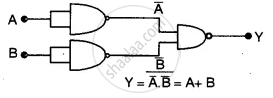# With the Help of a Diagram, Show How You Can Use Several Nand Gates to Obtain an Or Gate. - Physics (Theory)

Diagram

With the help of a diagram, show how you can use several NAND gates to obtain an OR gate.

Useful Constants and Relations :

 1. Speed of Light in Vacuum (c) = 3.00 x 108 m/s 2. Charge of a proton (e) = 1.60 x 10-19C 3. Planck's Constant (h) = 6.6 x 10-34 Js 4. Permeability of vacuum (μ0) = 4π x 10-7 Hm-1 5. Electron Volt (1eV ) = 1.6 x 10 6. Unified Atomic Mass Unit (1u) = 931 MeV (π) = 3.14 ( ln 2 ) = 0.693

#### Solution

In the figure is shown an ‘OR’ gate produced by combining ‘NAND’ gates only.Concept: Digital Electronics and Logic Gates
Is there an error in this question or solution?